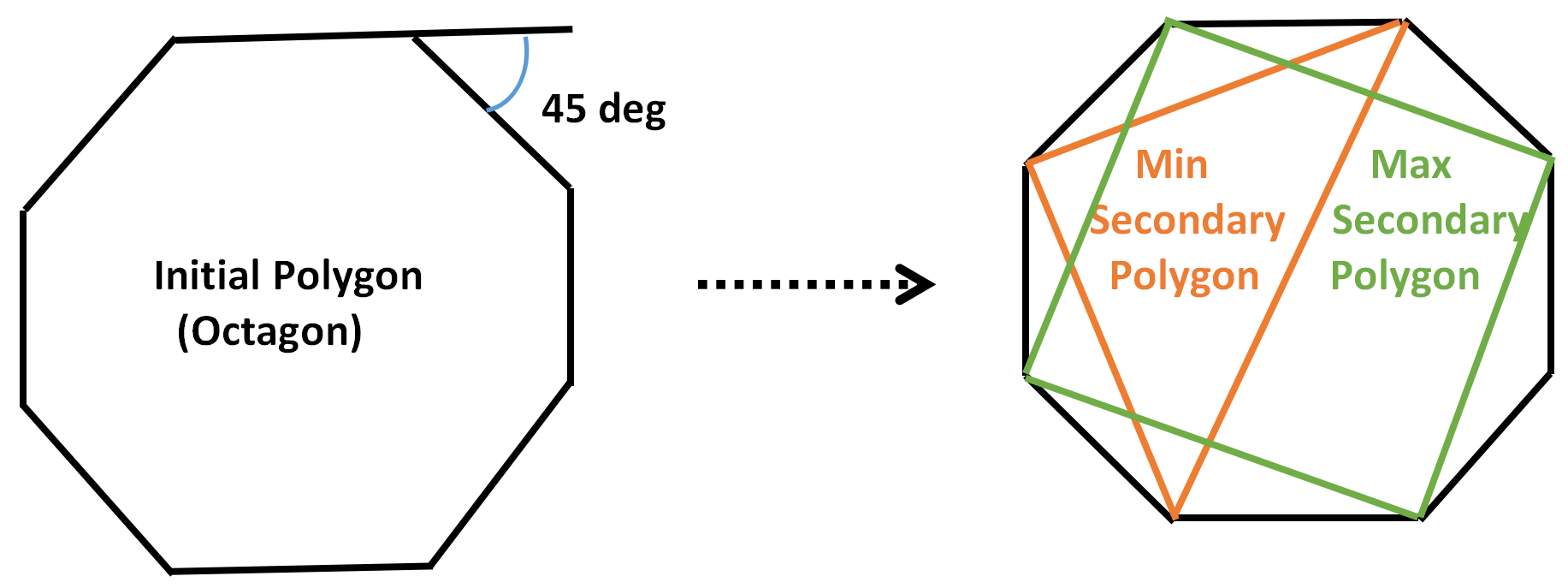Open In App

# Sum of sides of largest and smallest child polygons possible from a given polygon

Given an integer A representing the external angle (in degrees) of a regular convex polygon, the task is to find the sum of the sides of the largest and smallest secondary polygons formed such that each edge of the secondary polygon is a chord of the primary polygon. If it is not possible to form such polygons, then print “-1”.

Examples:

Input: A = 45
Output: 7
Explanation:
The primary polygon is an Octagon of 8 sides.
Therefore, the smallest secondary polygon consists of 3 edges and the largest secondary polygon consists of 4 edges.Sum of edges of the smallest secondary polygon + Edges of the largest secondary polygon = 3 + 4 = 7.

Input: A = 60
Output: 6
Explanation: The primary polygon is a Hexagon consisting of 6 sides. Therefore, the smallest secondary polygon consists of 3 edges and the largest secondary polygon consists of 3 edges.

Approach: The idea is to first find the number of edges in the primary polygon and then, check whether it is possible to make secondary polygons or not. Follow the steps below to solve the problem:

• The sum of the external angle in a regular polygon is 360 degrees. Therefore, the number of sides = 360 / external angle.
• The number of sides of the maximum secondary polygon is the number of sides of the primary polygon / 2.
• As polygon is possible if the count of edges is at least 3, a secondary polygon is possible if the edges of the initial polygon ? 6.
• The smallest possible polygon has 3 edges always.
• Print the sum of number of sides in both the largest and smallest polygons.

Below is the implementation of the above approach:

## C++

 `#include ``using` `namespace` `std;` `// Function to find the sum of largest and``// smallest secondary polygons if possible``void` `secondary_polygon(``int` `Angle)``{` `    ``// Count edges of primary polygon``    ``int` `edges_primary = 360/Angle;` `    ``if` `(edges_primary >= 6)``    ``{` `        ``// Calculate edges present in``        ``// the largest secondary polygon``        ``int` `edges_max_secondary = edges_primary / 2;` `        ``cout << edges_max_secondary + 3;``    ``}``    ``else``        ``cout << ``"Not Possible"``;``}` `// Driver Code``int` `main()``{``  ` `  ``// Given Exterior Angle``  ``int` `Angle = 45;``  ``secondary_polygon(Angle);` `  ``return` `0;``}` `// This code is contributed by mohit kumar 29.`

## Java

 `// Java program for the above approach``import` `java.io.*;``import` `java.util.*;``class` `GFG``{` `// Function to find the sum of largest and``// smallest secondary polygons if possible``static` `void` `secondary_polygon(``int` `Angle)``{` `    ``// Count edges of primary polygon``    ``int` `edges_primary = ``360``/Angle;` `    ``if` `(edges_primary >= ``6``)``    ``{` `        ``// Calculate edges present in``        ``// the largest secondary polygon``        ``int` `edges_max_secondary = edges_primary / ``2``;` `        ``System.out.println(edges_max_secondary + ``3``);``    ``}``    ``else``        ``System.out.println(``"Not Possible"``);``}`  `// Driver Code``public` `static` `void` `main(String[] args)``{``  ` `    ``// Given Exterior Angle``  ``int` `Angle = ``45``;``  ``secondary_polygon(Angle);``}``}` `// This code is contributed by code_hunt.`

## Python3

 `# Python3 program for the above approach:` `# Function to find the sum of largest and``# smallest secondary polygons if possible``def` `secondary_polygon(Angle):` `    ``# Count edges of primary polygon``    ``edges_primary ``=` `360``/``/``Angle` `    ``if` `edges_primary >``=` `6``:` `        ``# Calculate edges present in``        ``# the largest secondary polygon``        ``edges_max_secondary ``=` `edges_primary ``/``/` `2` `        ``return` `edges_max_secondary ``+` `3` `    ``else``:``        ``return` `"Not Possible"`  `# Driver Code``if` `__name__ ``=``=` `'__main__'``:` `    ``# Given Exterior Angle``    ``Angle ``=` `45``    ``print``(secondary_polygon(Angle))`

## C#

 `// C# program for the above approach``using` `System;``class` `GFG {` `  ``// Function to find the sum of largest and``  ``// smallest secondary polygons if possible``  ``static` `void` `secondary_polygon(``int` `Angle)``  ``{` `    ``// Count edges of primary polygon``    ``int` `edges_primary = 360 / Angle;` `    ``if` `(edges_primary >= 6) {` `      ``// Calculate edges present in``      ``// the largest secondary polygon``      ``int` `edges_max_secondary = edges_primary / 2;` `      ``Console.WriteLine(edges_max_secondary + 3);``    ``}``    ``else``      ``Console.WriteLine(``"Not Possible"``);``  ``}` `  ``// Driver Code``  ``public` `static` `void` `Main(``string``[] args)``  ``{` `    ``// Given Exterior Angle``    ``int` `Angle = 45;``    ``secondary_polygon(Angle);``  ``}``}` `// This code is contributed by ukasp.`

## Javascript

 ``

Output:

`7`

Time Complexity: O(1)
Auxiliary Space: O(1)